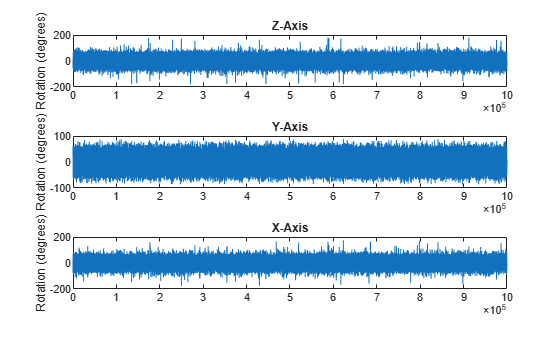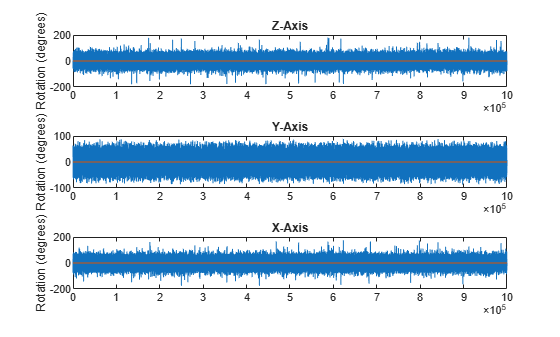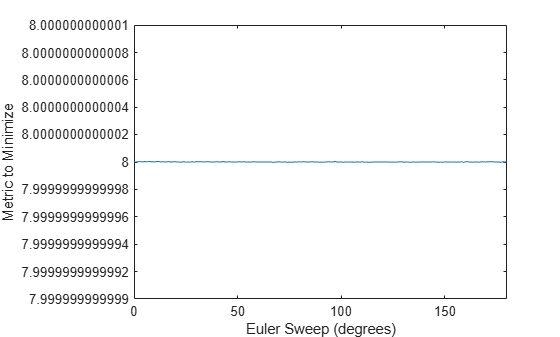Documentation

# meanrot

Quaternion mean rotation

## Syntax

``quatAverage = meanrot(quat)``
``quatAverage = meanrot(quat,dim)``
``quatAverage = meanrot(___,nanflag)``

## Description

example

````quatAverage = meanrot(quat)` returns the average rotation of the elements of `quat` along the first array dimension whose size not does equal 1.If `quat` is a vector, `meanrot(quat)` returns the average rotation of the elements.If `quat` is a matrix, `meanrot(quat)` returns a row vector containing the average rotation of each column.If `quat` is a multidimensional array, then `mearot(quat)` operates along the first array dimension whose size does not equal 1, treating the elements as vectors. This dimension becomes 1 while the sizes of all other dimensions remain the same.The `meanrot` function normalizes the input quaternions, `quat`, before calculating the mean.```
````quatAverage = meanrot(quat,dim)` return the average rotation along dimension `dim`. For example, if `quat` is a matrix, then `meanrot(quat,2)` is a column vector containing the mean of each row.```
````quatAverage = meanrot(___,nanflag)` specifies whether to include or omit `NaN` values from the calculation for any of the previous syntaxes. `meanrot(quat,'includenan')` includes all `NaN` values in the calculation while `mean(quat,'omitnan')` ignores them.```

## Examples

collapse all

Create a matrix of quaternions corresponding to three sets of Euler angles.

```eulerAngles = [40 20 10; ... 50 10 5; ... 45 70 1]; quat = quaternion(eulerAngles,'eulerd','ZYX','frame');```

Determine the average rotation represented by the quaternions. Convert the average rotation to Euler angles in degrees for readability.

`quatAverage = meanrot(quat)`
```quatAverage = quaternion 0.88863 - 0.062598i + 0.27822j + 0.35918k ```
`eulerAverage = eulerd(quatAverage,'ZYX','frame')`
```eulerAverage = 1×3 45.7876 32.6452 6.0407 ```

Use `meanrot` over a sequence of quaternions to average out additive noise.

Create a vector of 1e6 quaternions whose distance, as defined by the `dist` function, from quaternion(1,0,0,0) is normally distributed. Plot the Euler angles corresponding to the noisy quaternion vector.

```nrows = 1e6; ax = 2*rand(nrows,3) - 1; ax = ax./sqrt(sum(ax.^2,2)); ang = 0.5*randn(size(ax,1),1); q = quaternion(ax.*ang ,'rotvec'); noisyEulerAngles = eulerd(q,'ZYX','frame'); figure(1) subplot(3,1,1) plot(noisyEulerAngles(:,1)) title('Z-Axis') ylabel('Rotation (degrees)') hold on subplot(3,1,2) plot(noisyEulerAngles(:,2)) title('Y-Axis') ylabel('Rotation (degrees)') hold on subplot(3,1,3) plot(noisyEulerAngles(:,3)) title('X-Axis') ylabel('Rotation (degrees)') hold on```Use `meanrot` to determine the average quaternion given the vector of quaternions. Convert to Euler angles and plot the results.

```qAverage = meanrot(q); qAverageInEulerAngles = eulerd(qAverage,'ZYX','frame'); figure(1) subplot(3,1,1) plot(ones(nrows,1)*qAverageInEulerAngles(:,1)) title('Z-Axis') subplot(3,1,2) plot(ones(nrows,1)*qAverageInEulerAngles(:,2)) title('Y-Axis') subplot(3,1,3) plot(ones(nrows,1)*qAverageInEulerAngles(:,3)) title('X-Axis')```The `meanrot` Algorithm

The `meanrot` function outputs a quaternion that minimizes the squared Frobenius norm of the difference between rotation matrices. Consider two quaternions:

• `q0` represents no rotation.

• `q90` represents a 90 degree rotation about the x-axis.

```q0 = quaternion([0 0 0],'eulerd','ZYX','frame'); q90 = quaternion([0 0 90],'eulerd','ZYX','frame');```

Create a quaternion sweep, `qSweep`, that represents rotations from 0 to 180 degrees about the x-axis.

```eulerSweep = (0:1:180)'; qSweep = quaternion([zeros(numel(eulerSweep),2),eulerSweep], ... 'eulerd','ZYX','frame');```

Convert `q0`, `q90`, and `qSweep` to rotation matrices. In a loop, calculate the metric to minimize for each member of the quaternion sweep. Plot the results and return the value of the Euler sweep that corresponds to the minimum of the metric.

```r0 = rotmat(q0,'frame'); r90 = rotmat(q90,'frame'); rSweep = rotmat(qSweep,'frame'); metricToMinimize = zeros(size(rSweep,3),1); for i = 1:numel(qSweep) metricToMinimize(i) = norm((rSweep(:,:,i) - r0),'fro').^2 + ... norm((rSweep(:,:,i) - r90),'fro').^2; end plot(eulerSweep,metricToMinimize) xlabel('Euler Sweep (degrees)') ylabel('Metric to Minimize')``````[~,eulerIndex] = min(metricToMinimize); eulerSweep(eulerIndex)```
```ans = 45 ```

The minimum of the metric corresponds to the Euler angle sweep at 45 degrees. That is, `meanrot` defines the average between `quaterion([0 0 0],'ZYX','frame')` and `quaternion([0 0 90],'ZYX','frame')` as `quaternion([0 0 45],'ZYX','frame')`. Call `meanrot` with `q0` and `q90` to verify the same result.

`eulerd(meanrot([q0,q90]),'ZYX','frame')`
```ans = 1×3 0 0 45.0000 ```

Limitations

The metric that `meanrot` uses to determine the mean rotation is not unique for quaternions significantly far apart. Repeat the experiment above for quaternions that are separated by 180 degrees.

```q180 = quaternion([0 0 180],'eulerd','ZYX','frame'); r180 = rotmat(q180,'frame'); for i = 1:numel(qSweep) metricToMinimize(i) = norm((rSweep(:,:,i) - r0),'fro').^2 + ... norm((rSweep(:,:,i) - r180),'fro').^2; end plot(eulerSweep,metricToMinimize) xlabel('Euler Sweep (degrees)') ylabel('Metric to Minimize')``````[~,eulerIndex] = min(metricToMinimize); eulerSweep(eulerIndex)```
```ans = 159 ```

Quaternion means are usually calculated for rotations that are close to each other, which makes the edge case shown in this example unlikely in real-world applications. To average two quaternions that are significantly far apart, use the `slerp` function. Repeat the experiment using `slerp` and verify that the quaternion mean returned is more intuitive for large distances.

```qMean = slerp(q0,q180,0.5); q0_q180 = eulerd(qMean,'ZYX','frame')```
```q0_q180 = 1×3 0 0 90.0000 ```

## Input Arguments

collapse all

Quaternion for which to calculate the mean, specified as a scalar, vector, matrix, or multidimensional array of quaternions.

Data Types: `quaternion`

Dimension to operate along, specified as a positive integer scalar. If no value is specified, then the default is the first array dimension whose size does not equal 1.

Dimension `dim` indicates the dimension whose length reduces to 1. The `size(quatAverage,dim)` is `1`, while the sizes of all other dimensions remain the same.

Data Types: `double` | `single`

`NaN` condition, specified as one of these values:

• `'includenan'` –– Include `NaN` values when computing the mean rotation, resulting in `NaN`.

• `'omitnan'` –– Ignore all `NaN` values in the input.

Data Types: `char` | `string`

## Output Arguments

collapse all

Quaternion average rotation, returned as a scalar, vector, matrix, or multidimensional array.

Data Types: `single` | `double`

## Algorithms

`meanrot` determines a quaternion mean, $\overline{q}$, according to . $\overline{q}$ is the quaternion that minimizes the squared Frobenius norm of the difference between rotation matrices:

`$\overline{q}=\mathrm{arg}\begin{array}{c}\mathrm{min}\\ q\in {\text{S}}^{3}\end{array}\sum _{i=1}^{n}{‖A\left(q\right)-A\left({q}_{i}\right)‖}_{F}^{2}$`

 Markley, F. Landis, Yang Chen, John Lucas Crassidis, and Yaakov Oshman. "Average Quaternions." Journal of Guidance, Control, and Dynamics. Vol. 30, Issue 4, 2007, pp. 1193-1197.